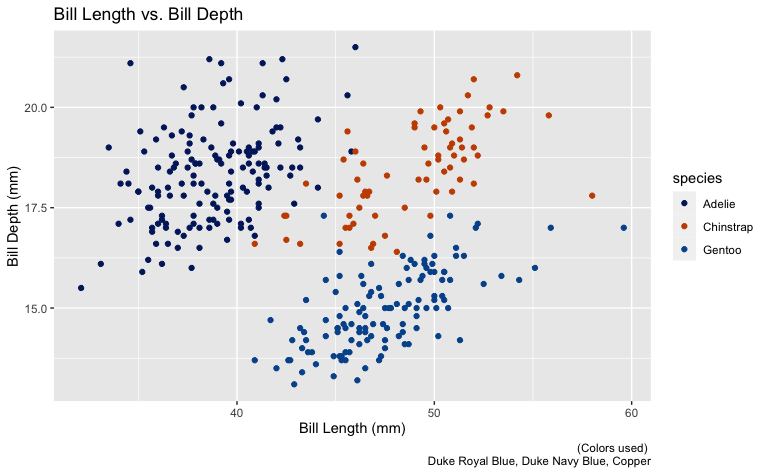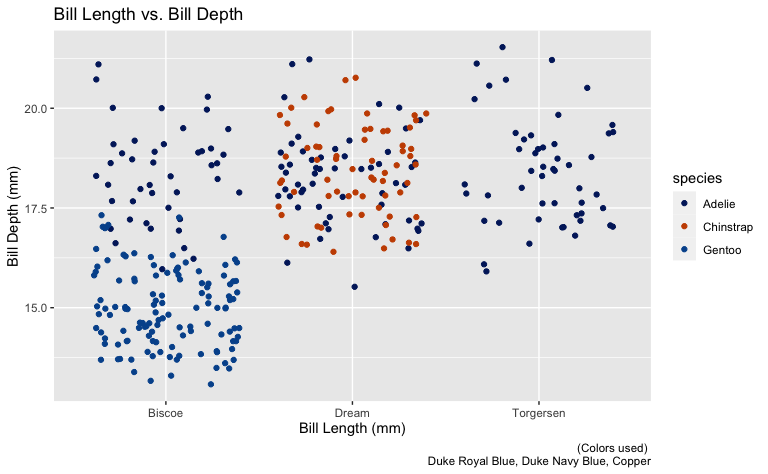# scale_duke_color_discrete()

This vignette is intended to demonstrate the functionality of scale_duke_color_discrete(). The function allows users to utilize a discrete color scale of Duke official branding colors on ggplot2 visualizations. It is fully integrated with ggplot2 visualizations.

# Plot Examples

For these visualizations, we will use the penguins dataset from the palmerpenguins package.

library(palmerpenguins)
library(duke)
library(ggplot2)
library(ggmosaic)

## Scatter Plot

plot <- ggplot(penguins, aes(x = bill_length_mm, y = bill_depth_mm)) +
geom_point(aes(color = species)) +
labs(
title = "Bill Length vs. Bill Depth",
caption = "(Colors used) \n Duke Royal Blue, Duke Navy Blue, Copper",
x = "Bill Length (mm)",
y = "Bill Depth (mm)"
)

plot +
scale_duke_color_discrete()## Jitter Plot

plot <- ggplot(penguins, aes(x = island, y = bill_depth_mm)) +
geom_jitter(aes(color = species)) +
labs(
title = "Bill Length vs. Bill Depth",
caption = "(Colors used) \n Duke Royal Blue, Duke Navy Blue, Copper",
x = "Bill Length (mm)",
y = "Bill Depth (mm)"
)

plot +
scale_duke_color_discrete()